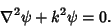## Poisson's Equation

A second-order Partial Differential Equation arising in physics:If, it reduces Laplace's Equation. It is also related to the Helmholtz Differential EquationArfken, G. Gauss's Law, Poisson's Equation.'' §1.14 in Mathematical Methods for Physicists, 3rd ed. Orlando, FL: Academic Press, pp. 74-78, 1985.